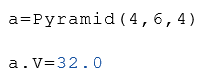# Function Pyramid

Calculate parameters of a pyramid

### Instruction

The Pyramid function calculates several properties of a pyramid.

The side length and the height are given as arguments. The number of corners can optionally be specified. If the number of corners is not specified, a pyramid with 4 corners is calculated.

The function returns a list with the following properties of the pyramid.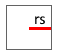rs Radius from the center to a straight line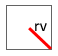rv Radius from the center to a corner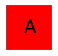A Area of the base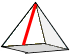L Height of one side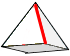e Length of an edge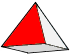S1 Surface of one side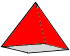S Surface without the base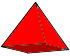Sg Total surface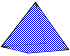V Volume

### Syntax

Pyramid (Width, Height)

Pyramid (Width, Heigth, Edges)

### Examples

Calculation of the properties from the side length $$a$$ and the height $$h$$.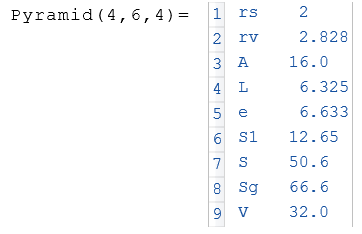In the example below, only the volume $$V$$ of the pyramid is displayed.